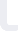Basic Statistics and Data Mining for Data Science Udemy

# Basic Statistics and Data Mining for Data Science

## Udemy

Price: USD 125
• Duration: Flexible

### Course details

Data science is an ever-evolving field, with exponentially growing popularity. Data science includes techniques and theories extracted from the fields of statistics, computer science, and most importantly machine learning, databases, and visualization.

This video course consists of step-by-step introductions to analyze data and the basics of statistics. The first chapter focuses on the steps to analyze data and which summary statistics are relevant given the type of data you are summarizing. The second chapter continues by focusing on summarizing individual variables and specifically some of the reasons users need to summarize variables. This chapter also illustrates several procedures, such as how to run and interpret frequencies and how to create various graphs. The third chapter introduces the idea of inferential statistics, probability, and hypothesis testing.

The rest of the chapters show you how to perform and interpret the results of basic statistical analyses (chi-square, independent and paired sample t-tests, one-way ANOVA, post-hoc tests, and bivariate correlations) and graphical displays (clustered bar charts, error bar charts, and scatterplots). You will also learn when to use different statistical techniques, how to set up different analyses, and how to interpret the results.